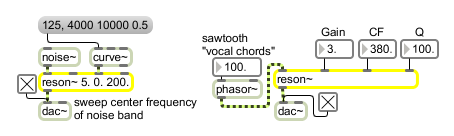# reson~

Resonant bandpass filter

## Description

reson~ implements the following filter equation:

y[n] = gain * (x[n] - r * x[n-2]) + c1 * y[n-1] + c2 * y[n-2], where r, c1, and c2 are parameters calculated from the input center-frequency and Q. Q is the filter-bandwidth divided by center-frequency. Inputs can be floats or signals.

## Arguments

Name Type Opt Description
initial-gain center-frequency and Q int or float opt Numbers set the initial gain, center frequency, and Q. The default values are 0 for gain, 0 for center frequency, and 0.01 for Q.

## Messages

 int initial-gain/center-frequency/Q [int] An int or float can be sent in the three right inlets to change the filter-gain, center-frequency, and Q. If a signal is connected to one of the inlets, a number received in that inlet is ignored. float initial-gain/center-frequency/Q [float] An int or float can be sent in the three right inlets to change the filter-gain, center-frequency, and Q. If a signal is connected to one of the inlets, a number received in that inlet is ignored. list initial-gain center-frequency and Q [list] The first number sets the filter-gain. The second number sets the filter center-frequency. The third number sets the filter-Q. If any of the inlets corresponding to these parameters have signals connected, the corresponding value in the list is ignored. clear Clears the filter's memory. Since reson~ is a recursive filter, this message may be necessary to recover from blowups. signal In left inlet: Any signal to be filtered. In left-middle inlet: Sets the bandpass filter gain. This value should generally be less than 1. In right-middle inlet: Sets the bandpass filter center frequency in hertz. In right inlet: Sets the bandpass filter "Q"-roughly, the sharpness of the filter - where Q is defined by the center-frequency divided by the filter-bandwidth. Useful Q values are typically between 0.01 and 500.

## Output

signal: The filtered input signal. The equation of the filter is

yn = gain * (xn - r * xn-2) + c1 * yn-1 + c2 * yn-2

where r, c1, and c2 are parameters calculated from the center frequency and Q.

## ExamplesControl gain center frequency and Q of a bandpass filter to alter a rich signal